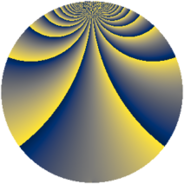# Properties

 Label 256.2.mLevel $256$ Weight $2$ Character orbit 256.m Rep. character $\chi_{256}(5,\cdot)$ Character field $\Q(\zeta_{64})$ Dimension $992$ Newform subspaces $1$ Sturm bound $64$ Trace bound $0$

# Related objects

## Defining parameters

 Level: $$N$$ $$=$$ $$256 = 2^{8}$$ Weight: $$k$$ $$=$$ $$2$$ Character orbit: $$[\chi]$$ $$=$$ 256.m (of order $$64$$ and degree $$32$$) Character conductor: $$\operatorname{cond}(\chi)$$ $$=$$ $$256$$ Character field: $$\Q(\zeta_{64})$$ Newform subspaces: $$1$$ Sturm bound: $$64$$ Trace bound: $$0$$

## Dimensions

The following table gives the dimensions of various subspaces of $$M_{2}(256, [\chi])$$.

Total New Old
Modular forms 1056 1056 0
Cusp forms 992 992 0
Eisenstein series 64 64 0

## Trace form

 $$992q - 32q^{2} - 32q^{3} - 32q^{4} - 32q^{5} - 32q^{6} - 32q^{7} - 32q^{8} - 32q^{9} + O(q^{10})$$ $$992q - 32q^{2} - 32q^{3} - 32q^{4} - 32q^{5} - 32q^{6} - 32q^{7} - 32q^{8} - 32q^{9} - 32q^{10} - 32q^{11} - 32q^{12} - 32q^{13} - 32q^{14} - 32q^{15} - 32q^{16} - 32q^{17} - 32q^{18} - 32q^{19} - 32q^{20} - 32q^{21} - 32q^{22} - 32q^{23} - 32q^{24} - 32q^{25} - 32q^{26} - 32q^{27} - 32q^{28} - 32q^{29} - 32q^{30} - 32q^{31} - 32q^{32} - 32q^{33} - 32q^{34} - 32q^{35} - 32q^{36} - 32q^{37} - 32q^{38} - 32q^{39} - 32q^{40} - 32q^{41} - 32q^{42} - 32q^{43} - 32q^{44} - 32q^{45} - 32q^{46} - 32q^{47} - 32q^{48} - 32q^{49} - 32q^{50} - 32q^{51} - 32q^{52} - 32q^{53} - 32q^{54} - 32q^{55} - 32q^{56} - 32q^{57} - 32q^{58} - 32q^{59} - 32q^{60} - 32q^{61} - 32q^{62} - 32q^{63} - 32q^{64} - 32q^{65} - 32q^{66} - 32q^{67} - 32q^{68} - 32q^{69} - 32q^{70} - 32q^{71} - 32q^{72} - 32q^{73} - 32q^{74} - 32q^{75} - 32q^{76} - 32q^{77} - 32q^{78} - 32q^{79} - 32q^{80} - 32q^{81} - 32q^{82} - 32q^{83} - 32q^{84} - 32q^{85} - 32q^{86} - 32q^{87} - 32q^{88} - 32q^{89} - 32q^{90} - 32q^{91} - 32q^{92} - 32q^{93} - 32q^{94} - 32q^{95} - 32q^{96} - 32q^{97} - 32q^{98} - 32q^{99} + O(q^{100})$$

## Decomposition of $$S_{2}^{\mathrm{new}}(256, [\chi])$$ into newform subspaces

Label Dim. $$A$$ Field CM Traces $q$-expansion
$$a_2$$ $$a_3$$ $$a_5$$ $$a_7$$
256.2.m.a $$992$$ $$2.044$$ None $$-32$$ $$-32$$ $$-32$$ $$-32$$